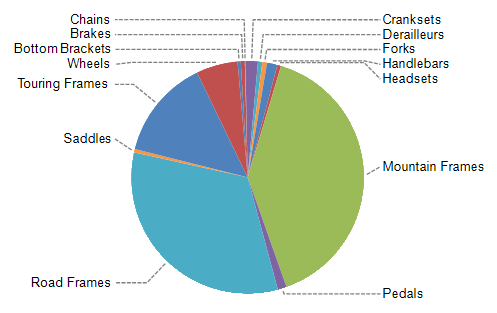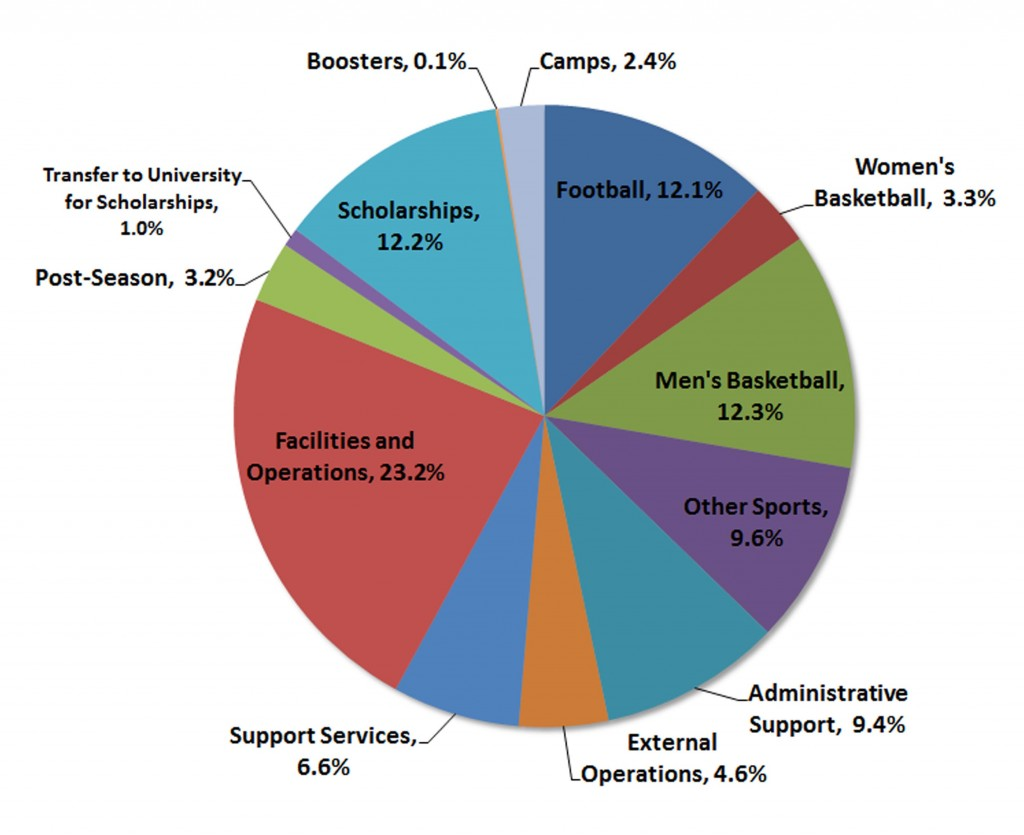## 极坐标系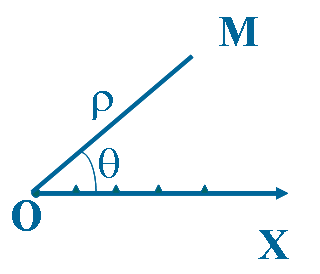## coord_polar

`coord_polar()` 是 `ggplot2` 中的极坐标函数，它可以弯曲横纵坐标，使用这个函数做出蜘蛛图或饼图的效果。我在网络上查了一下，比较少看到关于 `coord_polar()` 原理的介绍，只是在 `ggplot2` 的 Tidyverse 上发现了几个例子

```library(ggpubr)
library(ggplot2)

df <- data.frame(name = c("A", "B", "C"), value = c(10, 50, 30))
p <- ggplot(df, aes(x=name, y=value, fill=name)) + geom_bar(stat="identity", width=1, colour="black")
g <- ggplot(df, aes(x="", y=value, fill=name)) + geom_bar(stat="identity", width=1, colour="black")```

### 用法

`coord_polar()` 主要有四个参数： ​`theta`， ​`start`， ​`direction` 和 ​`clip` 。

`coord_polar(theta = "x", start = 0, direction = 1, clip = "on")`

• theta：variable to map angle to ( `x` or `y` ).
• start：offset of starting point from 12 o'clock in radians.
• direction：1, clockwise; -1, anticlockwise.
• clip：Should drawing be clipped to the extent of the plot panel? A setting of `"on"` (the default) means yes, and a setting of `"off"` means no.

1度=π/180≈0.01745弧度，1弧度=180/π≈57.3度。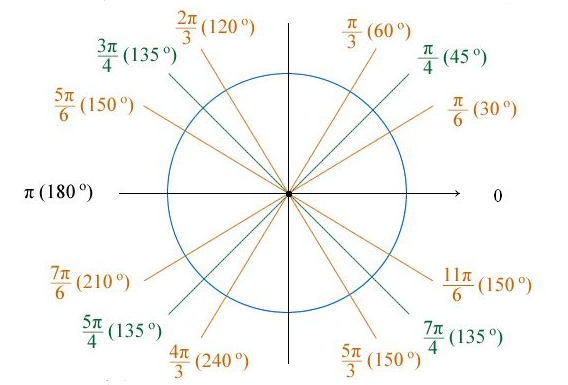### 参数示例

• theta="x"

x 轴极化，x 轴刻度值对应扇形弧度，y 轴刻度值对应圆环半径。p 中由于 x 是等长的，所以 p1 每一个弧度为 60 度；p2 的每一个弧度为 360 度。

```p1 <- p + coord_polar(theta="x") + labs(title="theta=\"x\"")
g1 <- g + coord_polar(theta="x") + labs(title="theta=\"x\"")
ggarrange(p, g, p1, g1, ncol=2, nrow=2, labels=c("p", "g", "p1", "g1"))```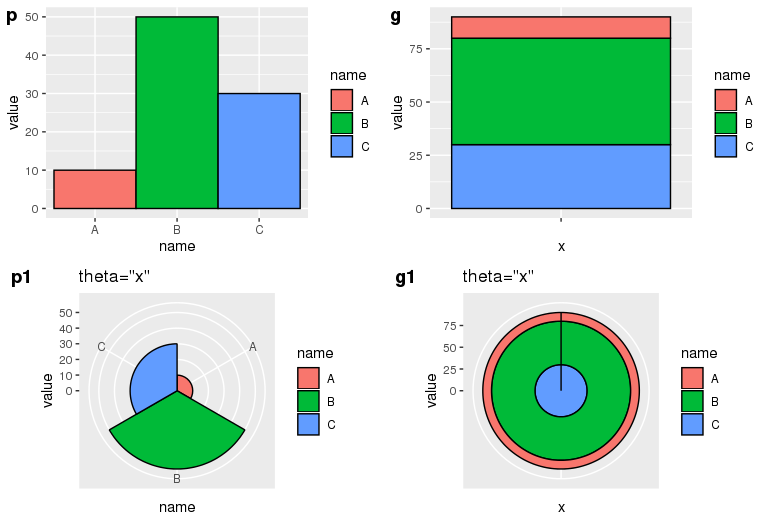• theta="y"

y 轴极化，y 轴刻度值对应扇形弧度，x 轴长度对应扇形半径。对于并列柱状图 p，以最大的 y 值作为 360 度的弧度，剩下的按比例类推，由于 p 中 A、B、C 是等长的，所以在 p1 中它们的半径是 1:2:3。对于堆叠柱状图 g，把 y 值按照比例划分弧度，因此它们的弧度比等于各自的 y 值比例。

```p2 <- p + coord_polar(theta="y") + labs(title="theta=\"y\"")
g2 <- g + coord_polar(theta="y") + labs(title="theta=\"y\"")
ggarrange(p, g, p2, g2, ncol=2, nrow=2, labels=c("p", "g", "p2", "g2"))```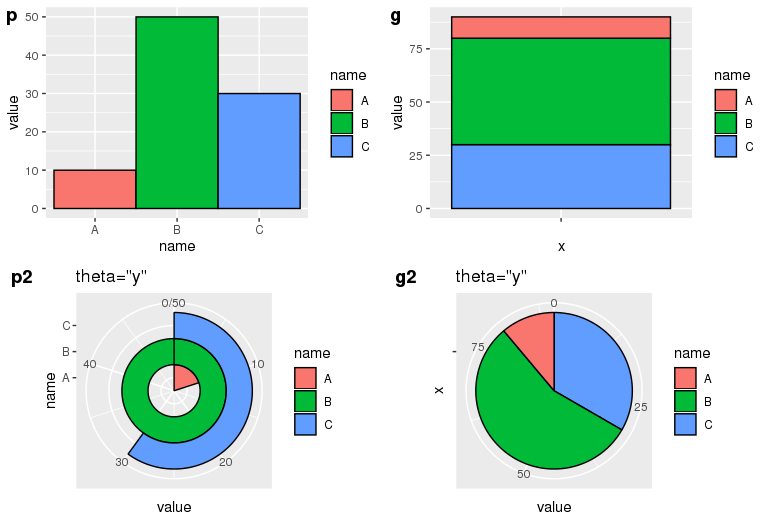• start=pi/6, direction=1

```p3 <- p + coord_polar(theta="y", start=pi/6, direction=1) + labs(title="theta=\"x\",start=pi/6,direction=1")
g3 <- g + coord_polar(theta="y", start=pi/6, direction=1) + labs(title="theta=\"x\",start=pi/6,direction=1")
ggarrange(p, g, p3, g3, ncol=2, nrow=2, labels=c("p", "g", "p3", "g3"))```• start=pi/6, direction=-1

```p4 <- p + coord_polar(theta="y", start=pi/6, direction=-1) + labs(title="theta=\"y\",start=pi/6,direction=-1")
g4 <- g + coord_polar(theta="y", start=pi/6, direction=-1) + labs(title="theta=\"y\",start=pi/6,direction=-1")
ggarrange(p, g, p4, g4, ncol=2, nrow=2, labels=c("p", "g", "p4", "g4"))```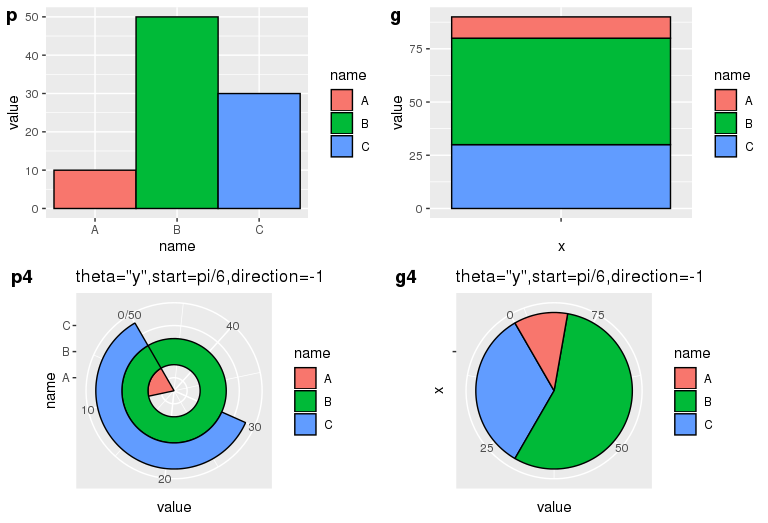• start=-pi/6, direction=1

```p5 <- p + coord_polar(theta="y", start=-pi/6, direction=1) + labs(title="theta=\"y\",start=-pi/6,direction=1")
g5 <- g + coord_polar(theta="y", start=-pi/6, direction=1) + labs(title="theta=\"y\",start=-pi/6,direction=1")
ggarrange(p, g, p5, g5, ncol=2, nrow=2, labels=c("p", "g", "p5", "g5"))```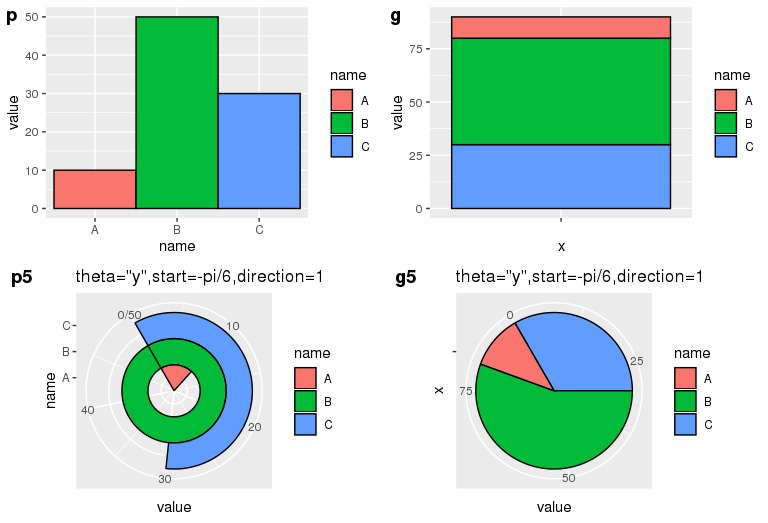Github 上有关于 coord-pola.r 的源码，整个代码只有 300 多行，有兴趣的同学可以去研究一下，上面的理解如有不对的地方还请看官们帮忙指正。

## 饼图中添加文字的位置控制 - 借助公式

```# Load ggplot2
library(ggplot2)

# Create Data
data <- data.frame(group=LETTERS[1:5], value=c(13,7,9,21,2))

# Basic piechart
ggplot(data, aes(x="", y=value, fill=group)) +
geom_bar(stat="identity", width=1) +
coord_polar("y", start=0)```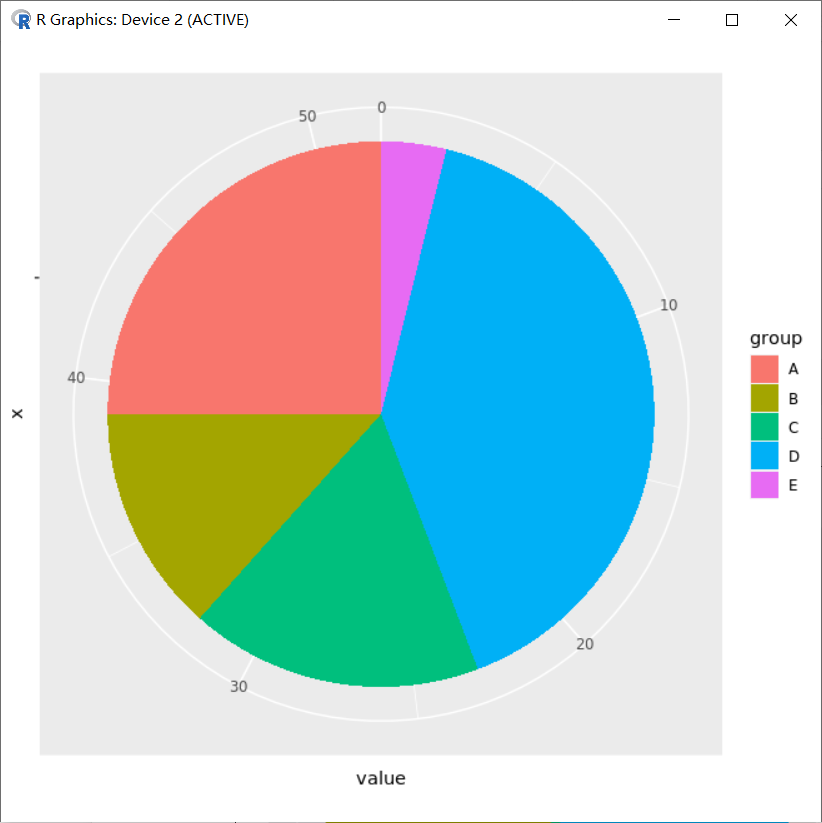```> dat <- data.frame(type=LETTERS[1:5], Num=c(90, 34, 56, 99, 15))
> dat
type Num
1    A  90
2    B  34
3    C  56
4    D  99
5    E  15```

```dat\$type <- factor(dat\$type,levels = dat\$type,order=T)
dat\$type```

``` A B C D E
Levels: A < B < C < D < E```

```p_pie <- ggplot(dat, aes(x="", y=dat[,2], fill=dat[,1]))+
geom_bar(stat="identity", width=1)+
coord_polar(theta="y", direction=1)+
scale_fill_brewer(palette ="Set3", direction = 1)+
labs(x="", y="", fill="Type")+
ggtitle(label ="test", subtitle=NULL)
p_pie```

scale_fill_brewer 是一个 ggplot2 和 RColorBrewer 关联的一个扩展调色板，其他可用于 scale_fill_brewer 调色板的颜色包括：

Diverging        BrBG, PiYG, PRGn, PuOr, RdBu, RdGy, RdYlBu, RdYlGn, Spectral

Qualitative      Accent, Dark2, Paired, Pastel1, Pastel2, Set1, Set2, Set3

Sequential       Blues, BuGn, BuPu, GnBu, Greens, Greys, Oranges, OrRd, PuBu, PuBuGn, PuRd, Purples,

RdPu, Reds, YlGn, YlGnBu, YlOrBr, YlOrRd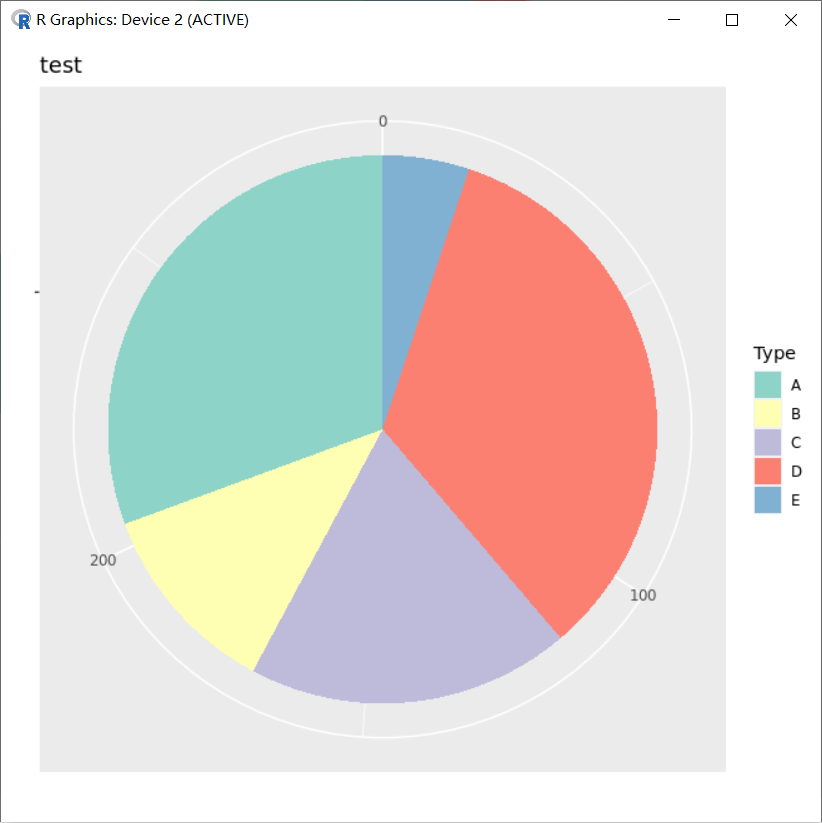```> sum(dat\$Num)-cumsum(dat\$Num)+dat\$Num/2
 249.0 187.0 142.0  64.5   7.5```

`cumsum` 是 R 语言 `base` 包 cum 系列的一个函数，它的功能是计算向量的累积和并返回。cum 系列还有另外三个函数： `cumprod``cummin``cummax` ，它们的作用分别是计算向量的累积的乘积、极小值、极大值，并返回。

```# 对数值型向量求和
> cumsum(1:10)
  1  3  6 10 15 21 28 36 45 55

# 对数值型矩阵求和，结果返回仍是向量
> cumsum(matrix(1:12, nrow = 3))
  1  3  6 10 15 21 28 36 45 55 66 78

# 对数据框求和，返回结果仍然是数据框，cumsum 会对对每个变量进行求和处理
> cumsum(data.frame(a = 1:10, b = 21:30))
a   b
1   1  21
2   3  43
3   6  66
4  10  90
5  15 115
6  21 141
7  28 168
8  36 196
9  45 225
10 55 255```

```p_pie=p_pie+
geom_text(aes(x=1.2,y=sum(dat\$Num)-cumsum(dat\$Num)+dat\$Num/2 ,label=as.character(dat[,2])),size=3)
p_pie```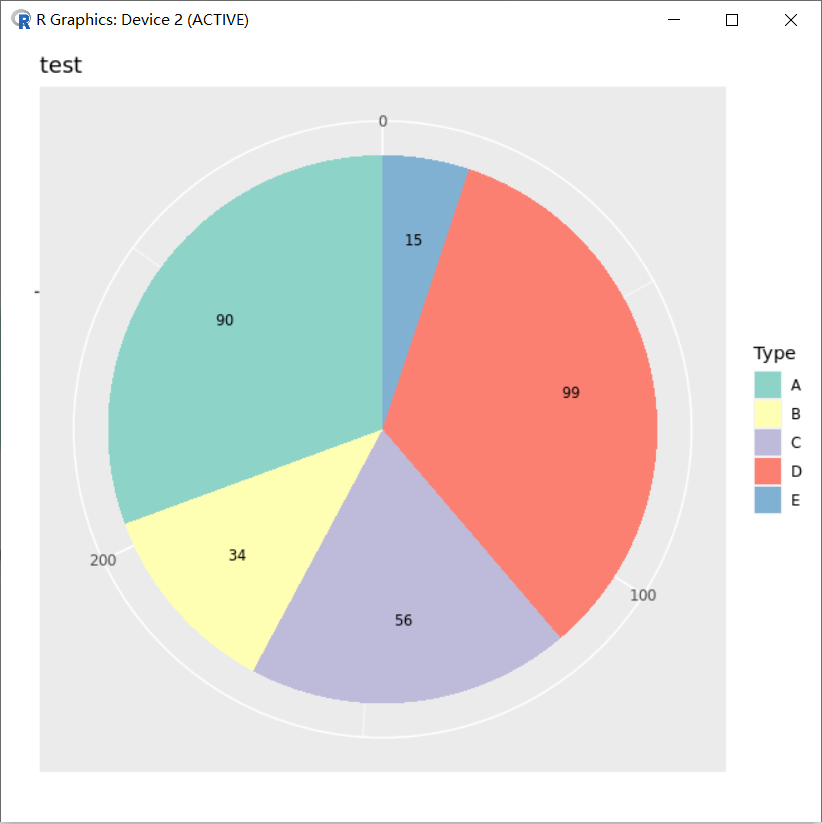```dat\$type=factor(dat\$type,levels = rev(dat\$type),order=T)
dat\$type

p_pie=ggplot(dat,aes(x="",y=dat[,2],fill=dat[,1]))+
geom_bar(stat="identity",width=1)+
coord_polar(theta="y",direction=1)+
scale_fill_brewer(palette ="Set3",direction = 1)+
labs(x="",y="",fill="Type")+
ggtitle(label ="test",subtitle=NULL)
p_pie```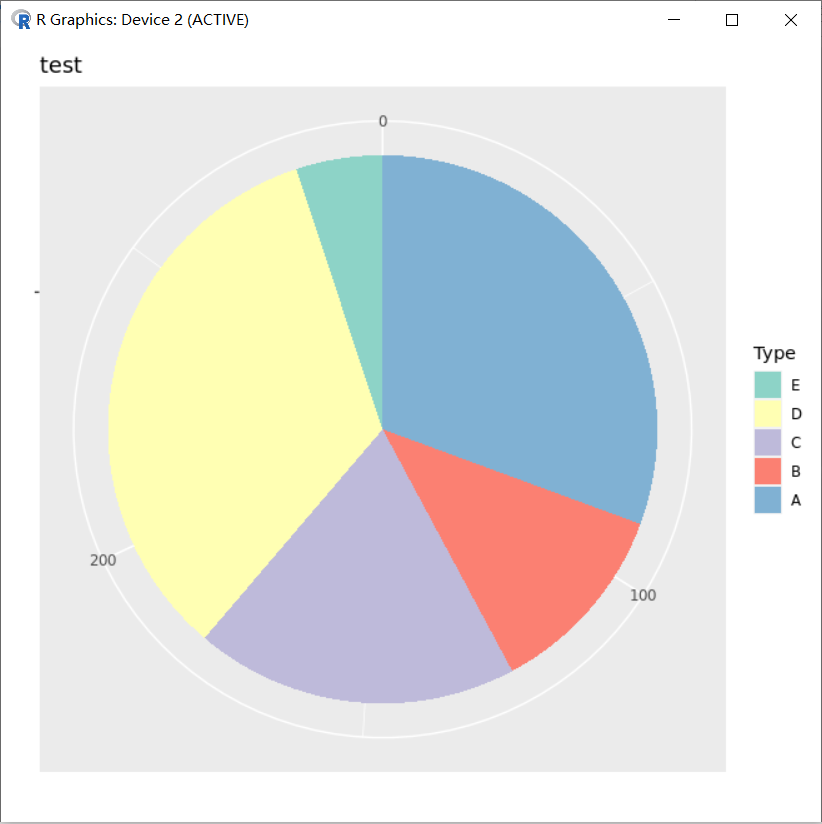```> cumsum(dat\$Num)-dat\$Num/2
  45.0 107.0 152.0 229.5 286.5```

```dat\$type=factor(dat\$type,levels = rev(dat\$type),order=T)
dat\$type

p_pie=ggplot(dat,aes(x="",y=dat[,2],fill=dat[,1]))+
geom_bar(stat="identity",width=1)+
coord_polar(theta="y",direction=1)+
scale_fill_brewer(palette ="Set3",direction = -1)+
labs(x="",y="",fill="Type")+
ggtitle(label ="test",subtitle=NULL)+
guides(fill=guide_legend(reverse = T))+
geom_text(aes(x=1.2,y=cumsum(dat\$Num)-dat\$Num/2 ,label=as.character(dat[,2])),size=3)

p_pie```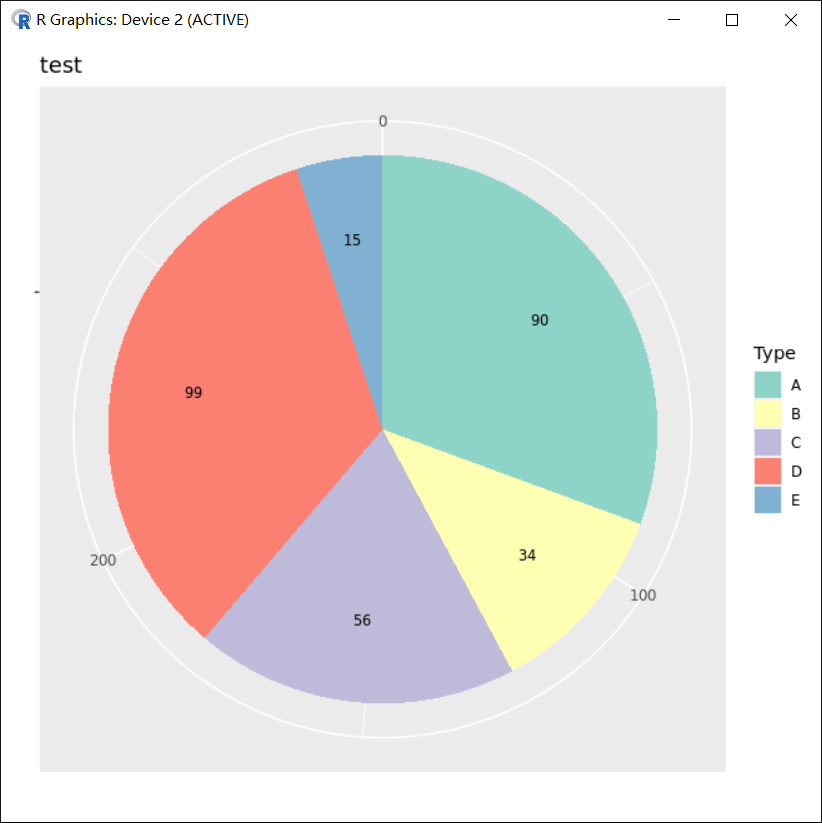## 饼图中添加文字的位置控制 - 非公式

• 数据

```> type <- c("A","B","C","D","E")
> Num <- c(90,34,56,99,15)
> dat <- data.frame(type,Num)
> dat
type Num
1    A  90
2    B  34
3    C  56
4    D  99
5    E  15```

• 创建有序因子，方便颜色填充
```> dat\$type <- factor(dat\$type,levels = dat\$type,order=T)
> dat\$type
 A B C D E
Levels: A < B < C < D < E```

• 画图，柱状图 position_stack(reverse =T) 控制填充顺序反向，第一个起始于坐标 0 的位置
```p_pie=ggplot(dat,aes(x="",y=dat[,2],fill=dat[,1]))+
geom_bar(stat="identity",width=1,position = position_stack(reverse =T))
p_pie```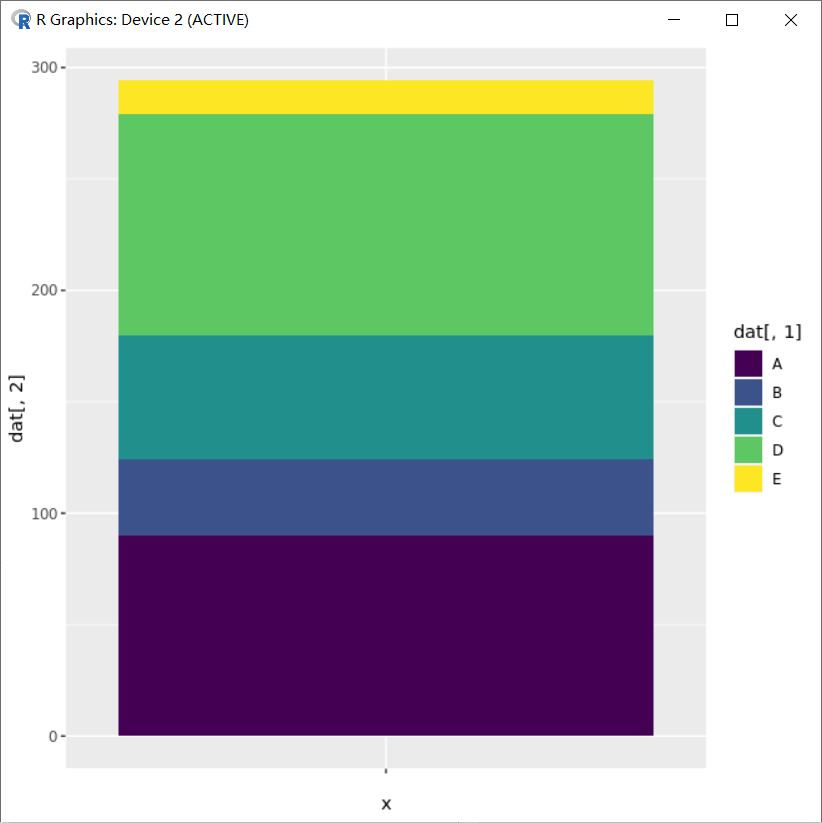• 极坐标变换与颜色等设置

```###添加极坐标进行图片变换
p_pie=p_pie+
coord_polar(theta="y",direction=1)
p_pie```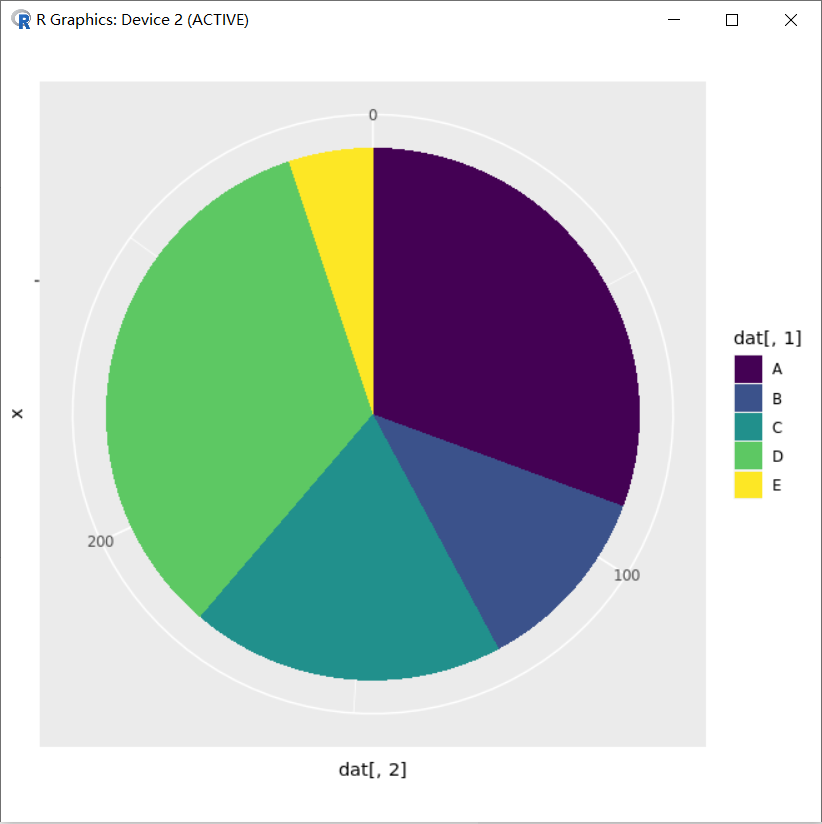```###修改颜色、标签
p_pie=p_pie+
scale_fill_brewer(palette ="Set3",direction = 1)+
labs(x="",y="",fill="Type")+
ggtitle(label ="test",subtitle=NULL)
p_pie```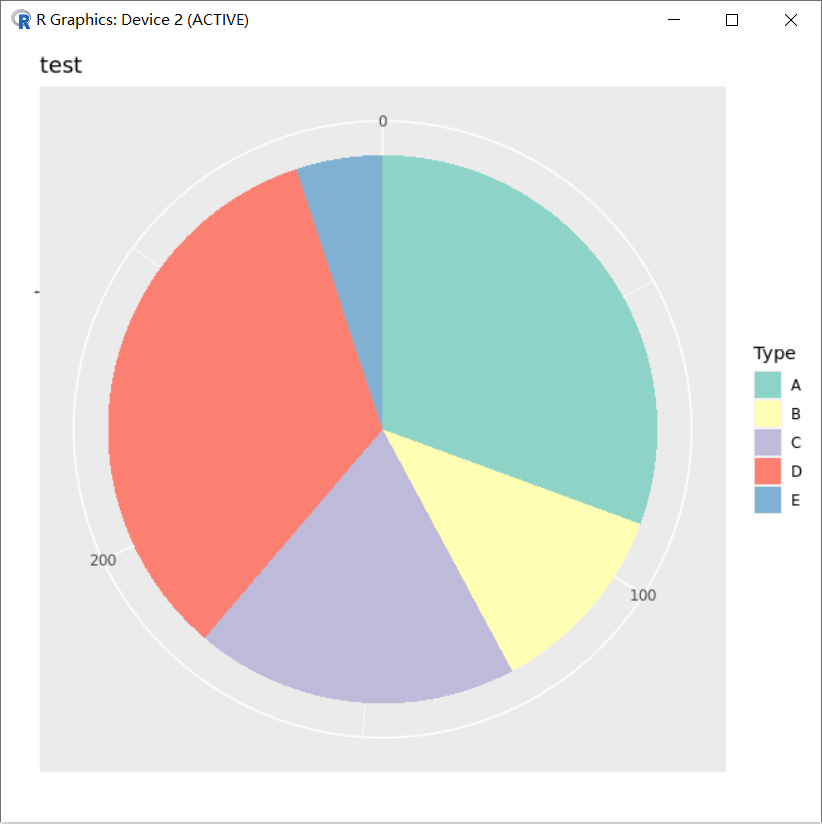• 控制 geom_text(position)

```### 添加文字，文字位置控制position = position_stack(reverse =T，vjust=0.5)
### reverse =T与柱子填充顺序同时反向，vjust=0.5在堆叠柱子的中间位置添加文字
p_pie=p_pie+
geom_text(aes(x=1.2,label=as.character(dat[,2])),position = position_stack(reverse =T,vjust=0.5),size=3)
p_pie```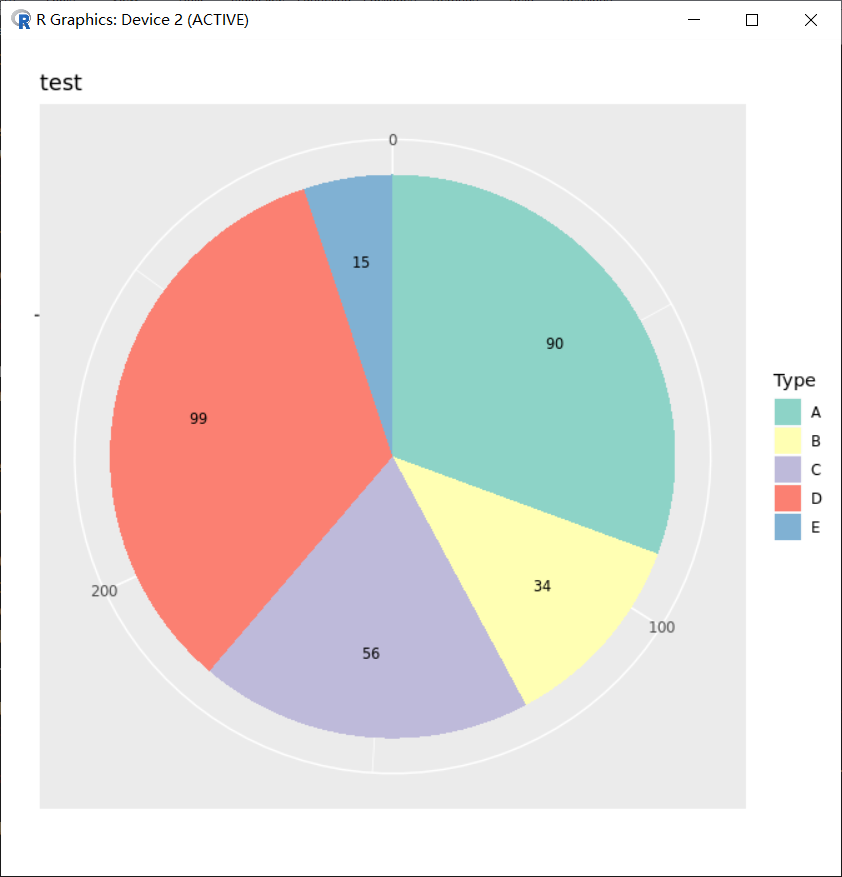## sessionInfo

```> sessionInfo()
R version 3.6.2 (2019-12-12)
Platform: x86_64-conda_cos6-linux-gnu (64-bit)
Running under: CentOS Linux 7 (Core)

Matrix products: default
BLAS/LAPACK: /usr/local/software/miniconda3/lib/libopenblasp-r0.3.8.so

locale:
 LC_CTYPE=en_US.UTF-8       LC_NUMERIC=C
 LC_TIME=en_US.UTF-8        LC_COLLATE=en_US.UTF-8
 LC_MONETARY=en_US.UTF-8    LC_MESSAGES=en_US.UTF-8
 LC_PAPER=en_US.UTF-8       LC_NAME=C
 LC_ADDRESS=C               LC_TELEPHONE=C
 LC_MEASUREMENT=en_US.UTF-8 LC_IDENTIFICATION=C

attached base packages:
 stats     graphics  grDevices utils     datasets  methods   base

other attached packages:
 ggplot2_3.2.1

loaded via a namespace (and not attached):
 Rcpp_1.0.3         viridisLite_0.3.0  digest_0.6.25      withr_2.1.2
 crayon_1.3.4       dplyr_0.8.3        assertthat_0.2.1   grid_3.6.2
 R6_2.4.0           gtable_0.3.0       magrittr_1.5       scales_1.0.0
 pillar_1.4.3       rlang_0.4.5        lazyeval_0.2.2     labeling_0.3
 RColorBrewer_1.1-2 glue_1.3.1         purrr_0.3.2        munsell_0.5.0
 compiler_3.6.2     pkgconfig_2.0.3    colorspace_1.4-1   tidyselect_0.2.5
 tibble_2.1.3
>```

## FAQ：如何实现 R 语言饼图标签的 overlap 问题？